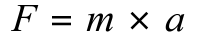# Knowledge questions on Forces, movement, shape and momentum for Edexcel IGCSE Physics

This topic is part of the Forces and Motion Topic

The specification can be found here.

1. Write down two forces. One begins with g and one begins with e.
2. What is the difference between a scalar and a vector?
4. Show by a diagram how three forces can be added together in a line.
5. Does friction oppose or help the motion of an object?
6. Write down what all the terms mean in his equation and rearrange it all ways →7. Write down the equation to calculate weight in terms of mass and gravitational field strength.
8. What is your understanding of gravitational field strength?
9. How do you calculate the stopping distance of a vehicle?
10. What factors affect the stopping distance of a vehicle?
11. Describe the forces acting on a falling object including reaching a terminal velocity.
12. Sketch a force-extension graph and label the part which represents Hooke’s Law.
13. What is Hooke’s Law?
14. What is elastic behaviour?
15. State the equation to calculate momentum. Write down the units and meaning of each term.
16. Use momentum to explain how crumple zones work and seat belts.
17. Write down the conservation of momentum.
18. What is Newton’s Third Law?
19. How can the moment of a force be calculated?
20. Where does the weight of an object act?
21. For balance what can you say about clockwise moments and anticlockwise moments?
22. Draw a diagram to show the upward forces on a light beam supported at each end as an object travels across it.

## You might also find useful

Online Tuition

Phone Physics Help